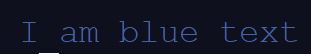The most useful python modules for beginners

Not long ago, I was a beginner to python. I was eager to make games, but had a hard time finding how to create certain elements of the game, such as choosing a random number or shuffling a list. That is when I discovered that there was no special tutorial in most places to teach you how to do these simple things. So thus, a tutorial by me (obviously) to help in things that don't have special tutorials.
(These are things I found useful)

#### CHOOSING A RANDOM NUMBER

To do this, at the top of your code, type in `import random`. This is what you call a module. Basically, a module is a bunch of functions that you can import to use in your code.

For example, if you want to make a variable called `x`, and assign in to a random number, you would do the following:
`x=random.randint(0,10)`

This is assigning a random integer or number between 0 and 10 to `x`.
So to create a random integer, first `import random`, then `randomvar=random.randint(start point, end point)`

So what ever random number you want, just remember the `random.randint()` part.

#### CHOOSING A RANDOM ITEM FROM A LIST

To do this, instead of typing `random.randint()`, you type `random.choice(thenameofyourlist)`.

For example, if I had a list like this:
`fruit=['apple','banana','orange']`

And I wanted to assign assign a variable called `y` to a random item from the `fruit` list, I would type the following:
`y=random.choice(fruit)`

#### SHUFFLING A LIST

If I wanted to shuffle `fruit`, just do the following:
`random.shuffle(fruit)`

#### WAITING A FEW SECONDS

For this, `import time`. Now if we want to wait between two `print` statements, we would do the following:
`print('hi')`
`time.sleep(1)`
`print('bye')`

In this case, it will print 'hi', wait 1 second, then print 'bye'.

PS- I made it wait only 1 second, but it can sleep for as long as you want by entering a different number in the `time.sleep()` function.

#### CLEARING THE TERMINAL

For this, `import os` then type `os.system('clear')`. This will clear the terminal.

#### COLOURS IN PYTHON

Thanks to @Bookie0 for reminding me to add colours
I wanted to to do this a lot when I started out with python, but I couldn't find anywhere that told me how to print coloured text. But after a little snooping around in other people's repls when posted, figured it out.

``````red = "\033[0;31m"
green = "\033[0;32m"
yellow = "\033[0;33m"
blue = "\033[0;34m"
magenta = "\033[0;35m"
cyan = "\033[0;36m"
white = "\033[0;37m"
bright_black = "\033[0;90m"
bright_red = "\033[0;91m"
bright_green = "\033[0;92m"
bright_yellow = "\033[0;93m"
bright_blue = "\033[0;94m"
bright_magenta = "\033[0;95m"
bright_cyan = "\033[0;96m"
bright_white = "\033[0;97m"``````

This is a list of colour values in python (copy and paste them into your code). To do an example, let's `print('I am blue text')` in blue (obviously). To `print` this in blue, it is similar to printing a variable with some text. We would do
`print(Blue,'I am blue text')`We can use any colour or effect we want, from the above list.

Below are some additional effects and ways you can `print` your text (they all work in the way shown above.

``````underline = "\033[4m"
italic = "\033[3m"
darken = "\033[2m"
invisible='\033[08m'
reverse='\033[07m'
reset='\033[0m'``````

Feel free to experiment with the colours and different types of text decoration.

#### THE END

Hope you liked it :)